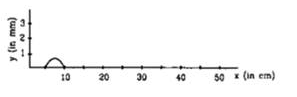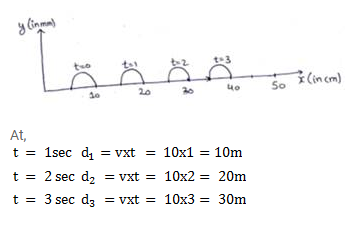# The Figure shows a wave pulse at t=0.`
Question:

The Figure shows a wave pulse at $t=0$. The pulse moves to the right with a speed at $10 \mathrm{~cm} / \mathrm{s}$. Sketch the shape of the string at $t=1 \mathrm{~s}, 2 \mathrm{~s}, 3 \mathrm{~s}$.Solution: